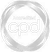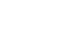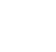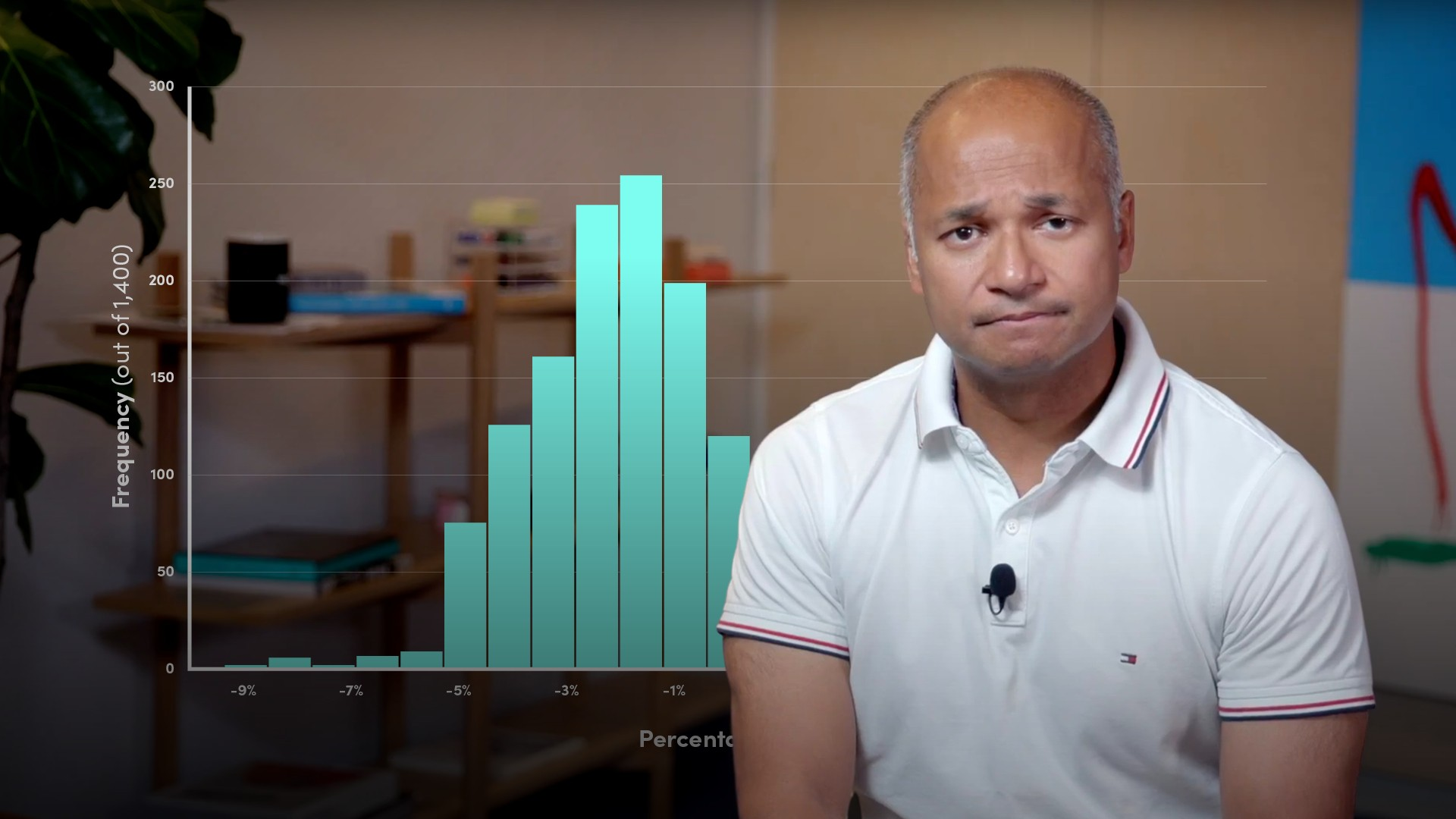# Value-at-Risk (VaR) Introduction25 years: Treasury & ALM

Any transaction undertaken by a bank carries an element of risk. Value-at-risk, or VaR, quantifies the probability of loss to a dollar value. Moorad shows how this is achieved using the variance-covariance method.

Financial Maths

Risk management

Treasury OperationsAny transaction undertaken by a bank carries an element of risk. Value-at-risk, or VaR, quantifies the probability of loss to a dollar value. Moorad shows how this is achieved using the variance-covariance method.

Finance Unlocked is the video learning platform built for finance professionals.### Value-at-Risk (VaR) Introduction

15 mins 25 secs

Overview

Value-at-Risk (VaR) is a methodology used to estimate the risk exposure of assets such as equities, bonds, or loans, within a specific time horizon and confidence level. VaR measures the volatility of the change in value of a bank’s specified balance sheet assets, and so the greater the volatility, the higher the probability of loss. It quantifies the potential loss in market value of a portfolio. The different VaR methodologies are correlation method, historical simulation method, and the Monte Carlo simulation method. To calculate the VaR using the variance-covariance method, you need to determine the time horizon over which the firm wishes to estimate a potential loss, select the confidence level, create a probability distribution of likely returns for the relevant investment, and then finally calculate the VaR estimate.

Key learning objectives:

• Define VaR

• Outline the main VaR methodologies

• Learn how to calculate VaR using the variance-covariance method

## Book a demo to access

Summary

#### What is risk, and what are the types of risk a bank faces?

• Risk - The uncertainty of the future total cash value of an investment on the investor’s horizon date
• Market Risk - The risk arising from movements in prices in financial markets. Examples include FX risk, interest rate risk and basis risk
• Credit Risk - Also referred to as default risk or counterparty risk, refers to risk that a consumer to whom a bank has lent money, will default on the loan

#### What is Value-at-Risk (VaR)?

• VaR measures the volatility of the change in value of a bank’s specified balance sheet assets, and so the greater the volatility the higher the probability of loss
• It is a measurement of market risk or credit risk. It is the maximum loss that can occur, stated to a degree of confidence, say X%, over a period of specified holding period of t days
• So, for example if a daily VaR was calculated to be £10,000 to a 95% level of confidence, this means that during the day there is a 5% chance that the loss will be greater than £100,000

#### What are some common misconceptions about VaR?

• It is a unified method for measuring risk
• Measures other risks that a bank will be exposed to
• It is the maximum amount of money that a balance sheet position can lose

#### What are the main VaR methodologies?

• The correlation method
• The historical simulation method
• The Monte Carlo simulation

#### What are the assumptions behind the correlation method?

• Assumes that the returns on risk factors are normally distributed
• Assumes the correlations between risk factors are constant
• Assumes the delta of each portfolio constituent is constant
• Volatility of each risk factor is extracted from the historical observation period

#### How do we calculate the relevant risk factors?

1. Simple historic volatility (correlation) - However, the effects of a large one-off market move can significantly distort volatilities over the required forecasting period
2. Weighting past observations unequally - This is done to give more weight to recent observations so that large jumps in volatility are not caused by events long ago

#### What does the historical simulation method consist of?

• The simplest method and avoids some of the pitfalls of the correlation method
• The model calculates potential losses using actual historical returns in the risk factors
• Captures the non-normal distribution of risk factor returns
• As the risk factor returns used for revaluing the portfolio are actual past movements, the correlations in the calculation are also past correlations
• Runs a portfolio through actual historical price data to create a hypothetical time series of returns in estimating VaR

#### What are the pros and cons of the Monte Carlo method?

• More flexible than the correlation method and the historical simulation method
• Allows the risk manager to use actual historical distributions for risk factor returns
• A large number of randomly generated simulations are then run forward in time using volatility and correlation estimates
• However, its implementation requires greater processing power, and there may be a trade-off in that the time taken to perform calculations is longer

#### How do we calculate VaR using the variance-covariance method?

1. Determine the time horizon over which the firm wishes to estimate a potential loss
• Time horizons of one day to one year have been used
• For a trading book, it may be a one-day period
• Regulators and participants in illiquid markets may want to estimate exposures to market risk over a longer period
2. Select the degree of certainty required
• The largest likely loss a bank will suffer 95 times out of 100, or 1 working day out of 20 (95% confidence interval) may be sufficient
• For regulatory requirements and senior management/shareholders, a 99% confidence interval may be more appropriate
3. Create a probability distribution of likely returns for the instrument or portfolio under consideration
• The easiest to understand is a distribution of recent historical returns for the asset or portfolio
4. Calculating the VaR estimate
• Value the current portfolio using today’s prices
• Revalue the portfolio using alternative prices based on changed market factors and calculate the change in the portfolio value that would result
• Revaluing the portfolio using a number of alternative prices gives a distribution of changes in value. Given this, a portfolio VaR can be specified in terms of confidence levels
• The risk manager can then calculate the maximum the firm can lose over a specified time horizon at a specified probability level

#### What are the pros and cons of the variance-covariance method?

1. Advantages - It is simple to apply, and fairly straightforward to explain; datasets for its use are immediately available
2. Disadvantages - It assumes stable correlations and measures only linear risk; it also places excessive reliance on the normal distribution

## Book a demo to access

ExpertFormer senior banker with almost 30 years experience. Moorad has held senior roles in commerical, investment and retail banking. He has also spent the last five years as a visiting professor.09:58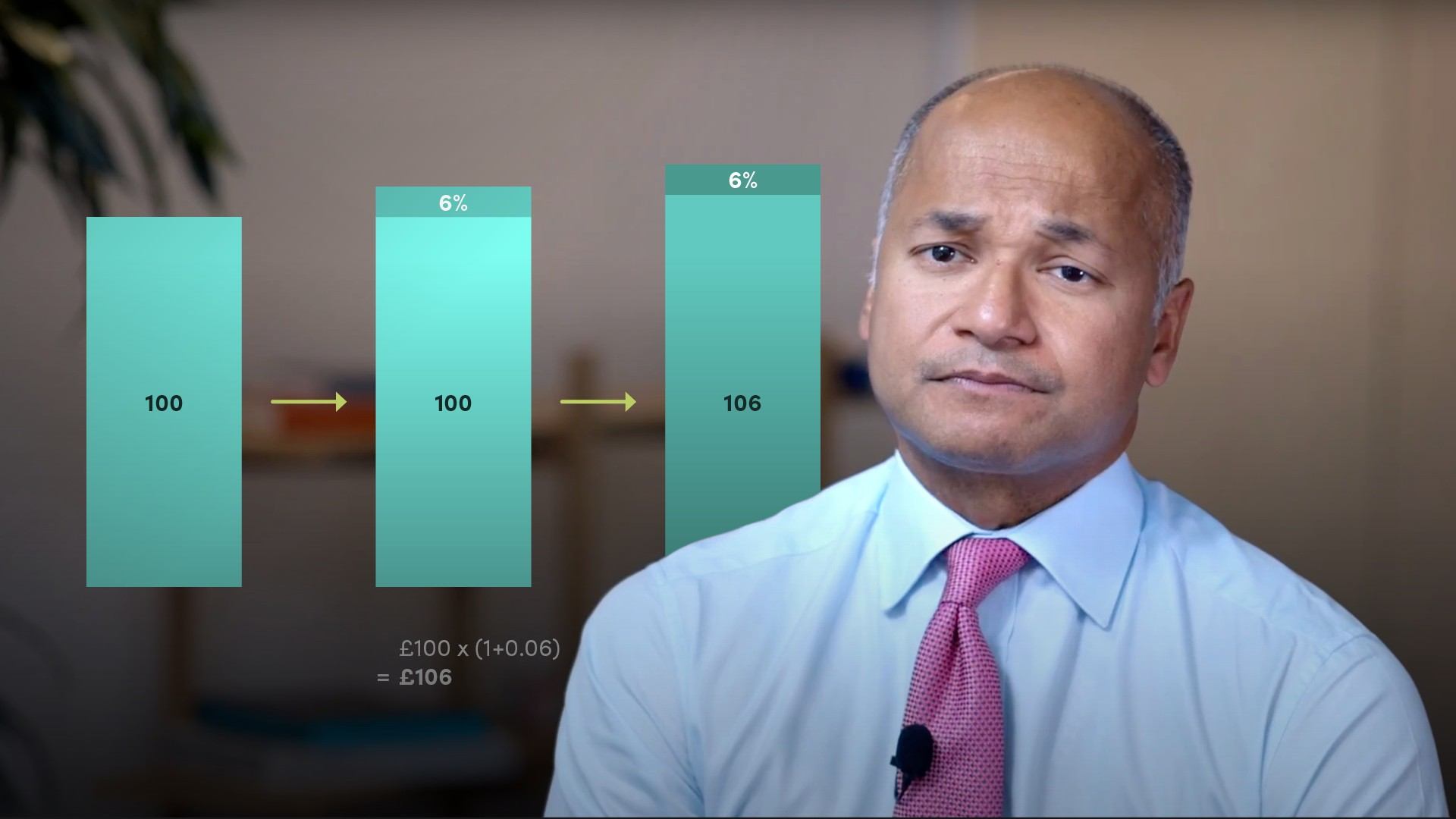09:4706:2605:22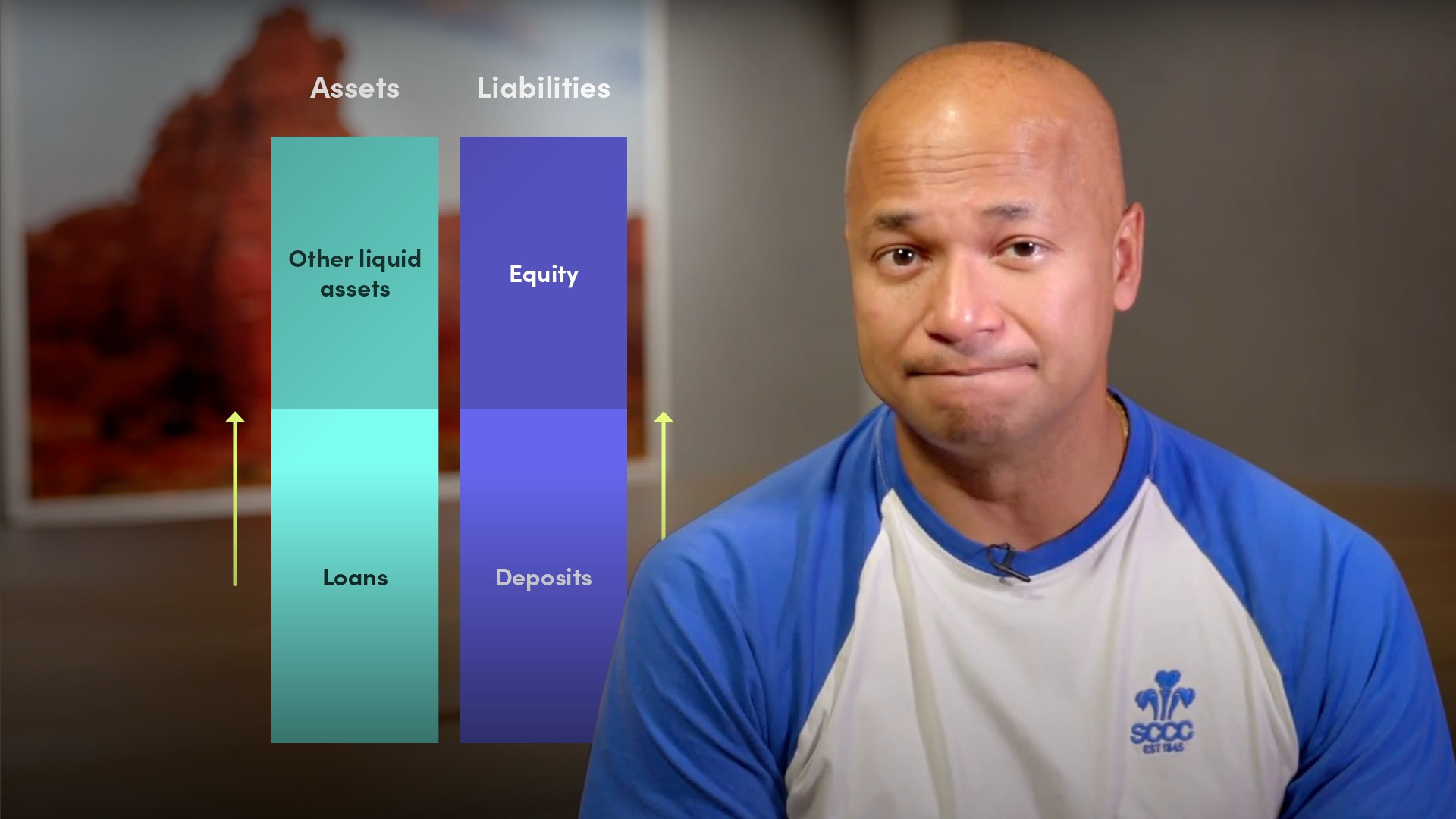03:26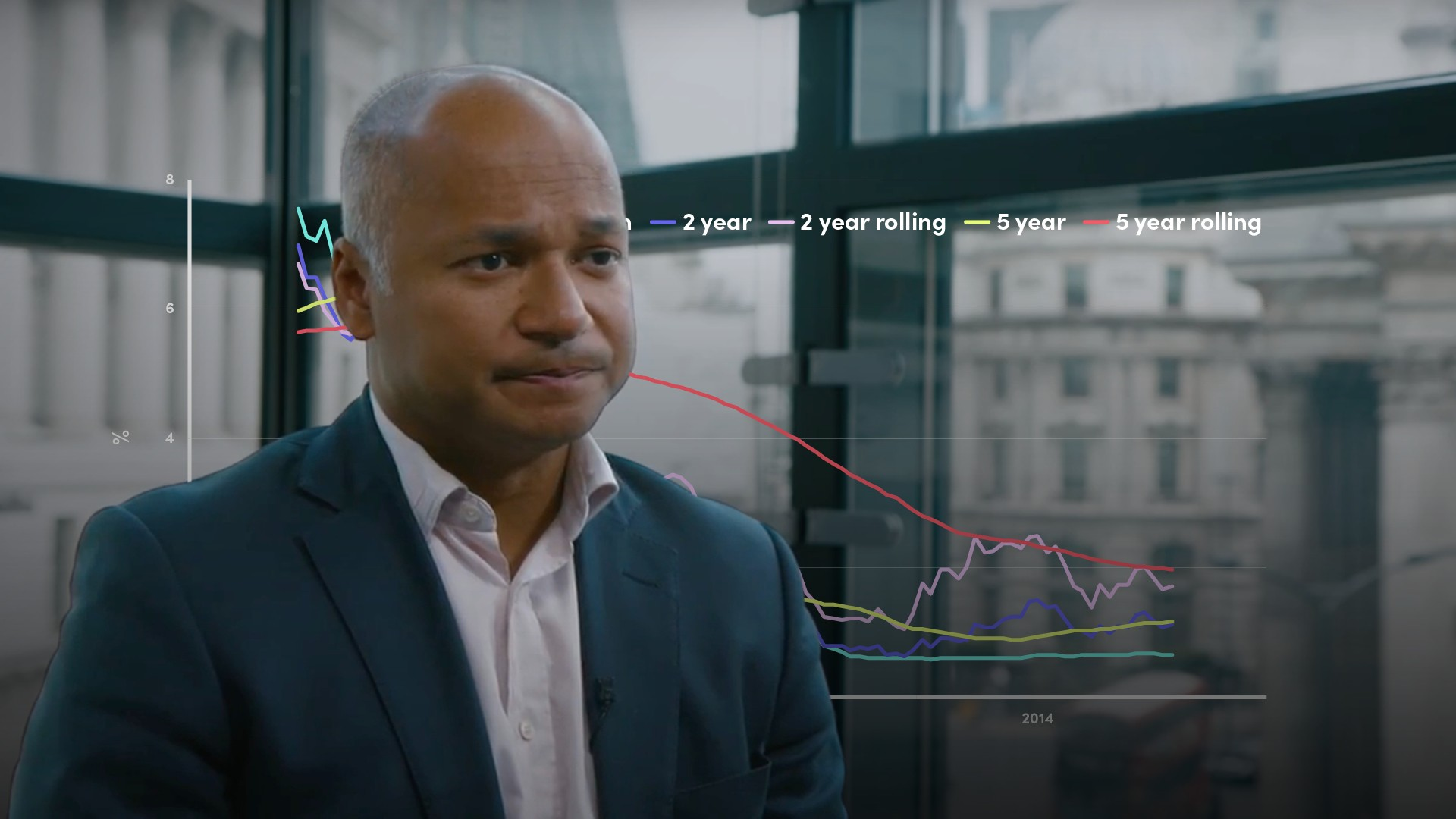14:5311:28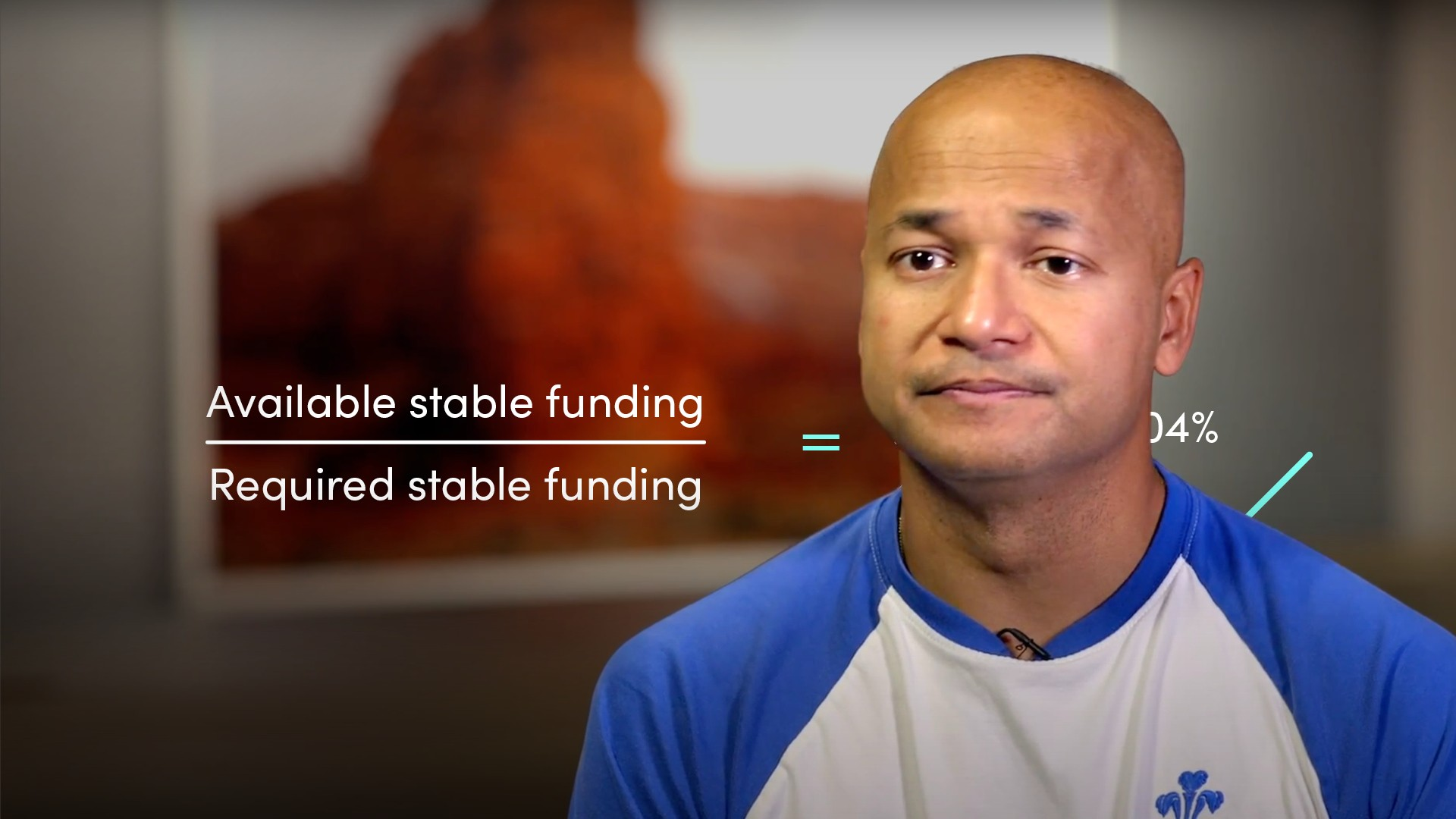07:3513:19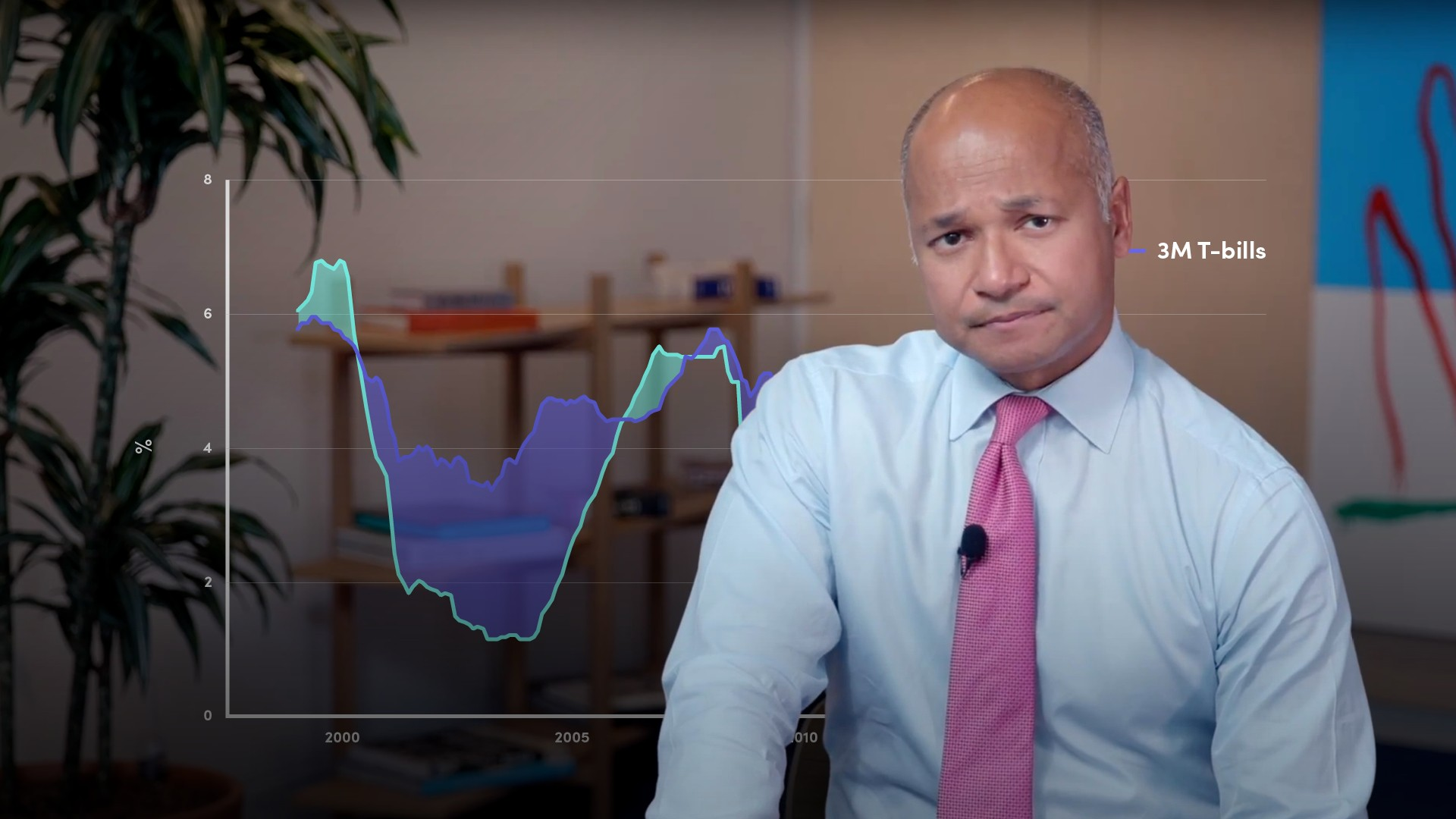11:47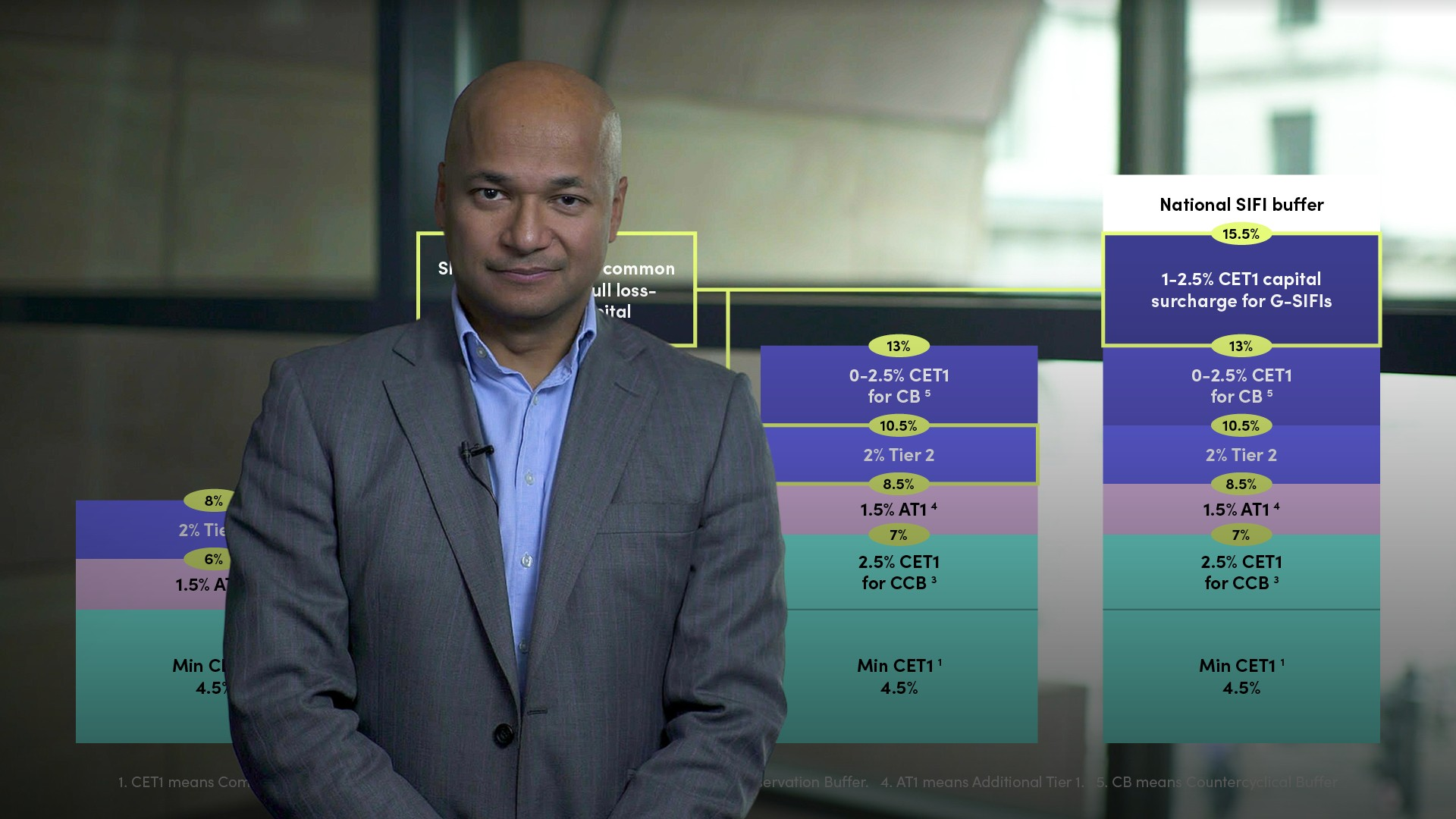11:0725:0919:44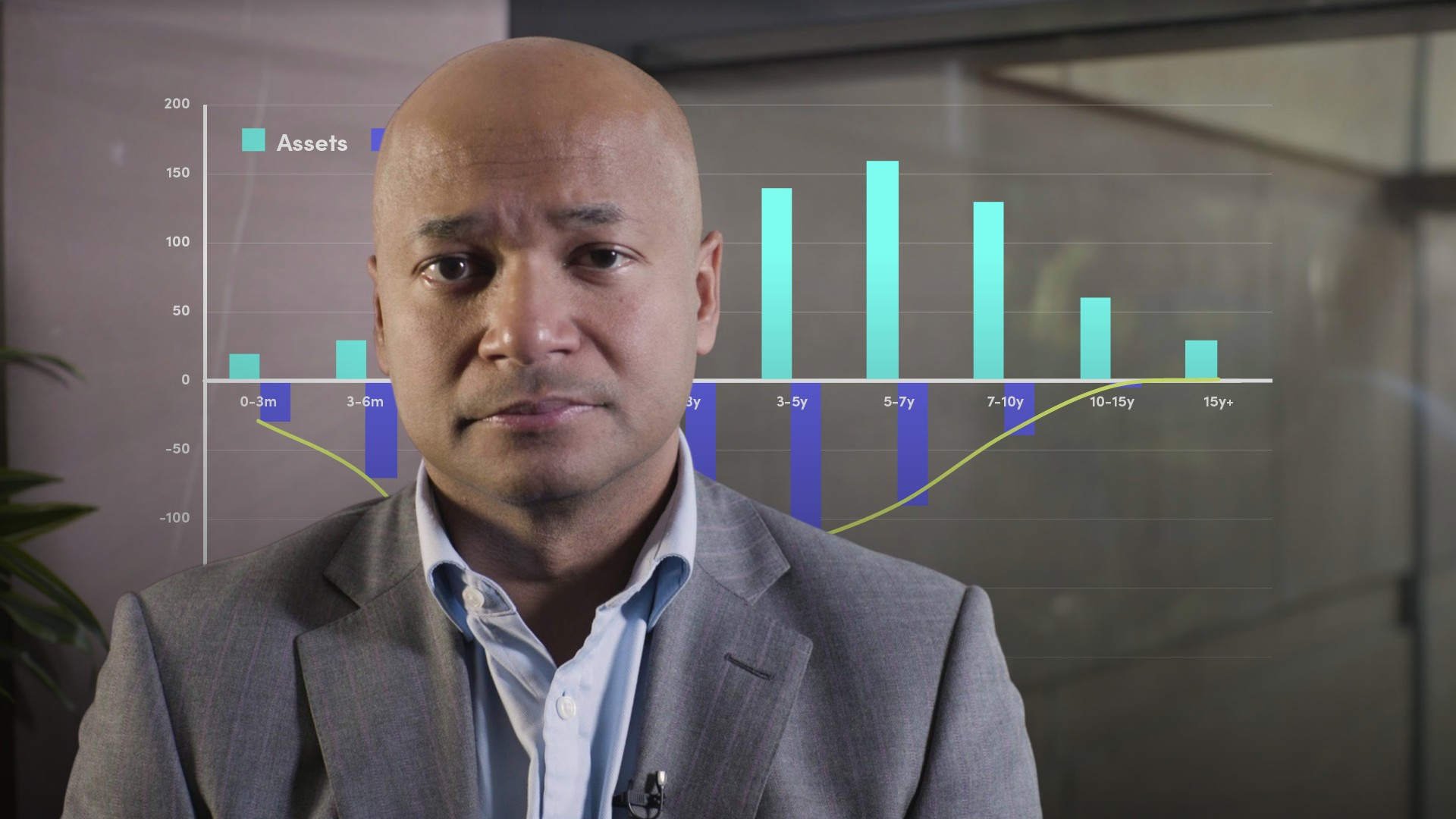17:04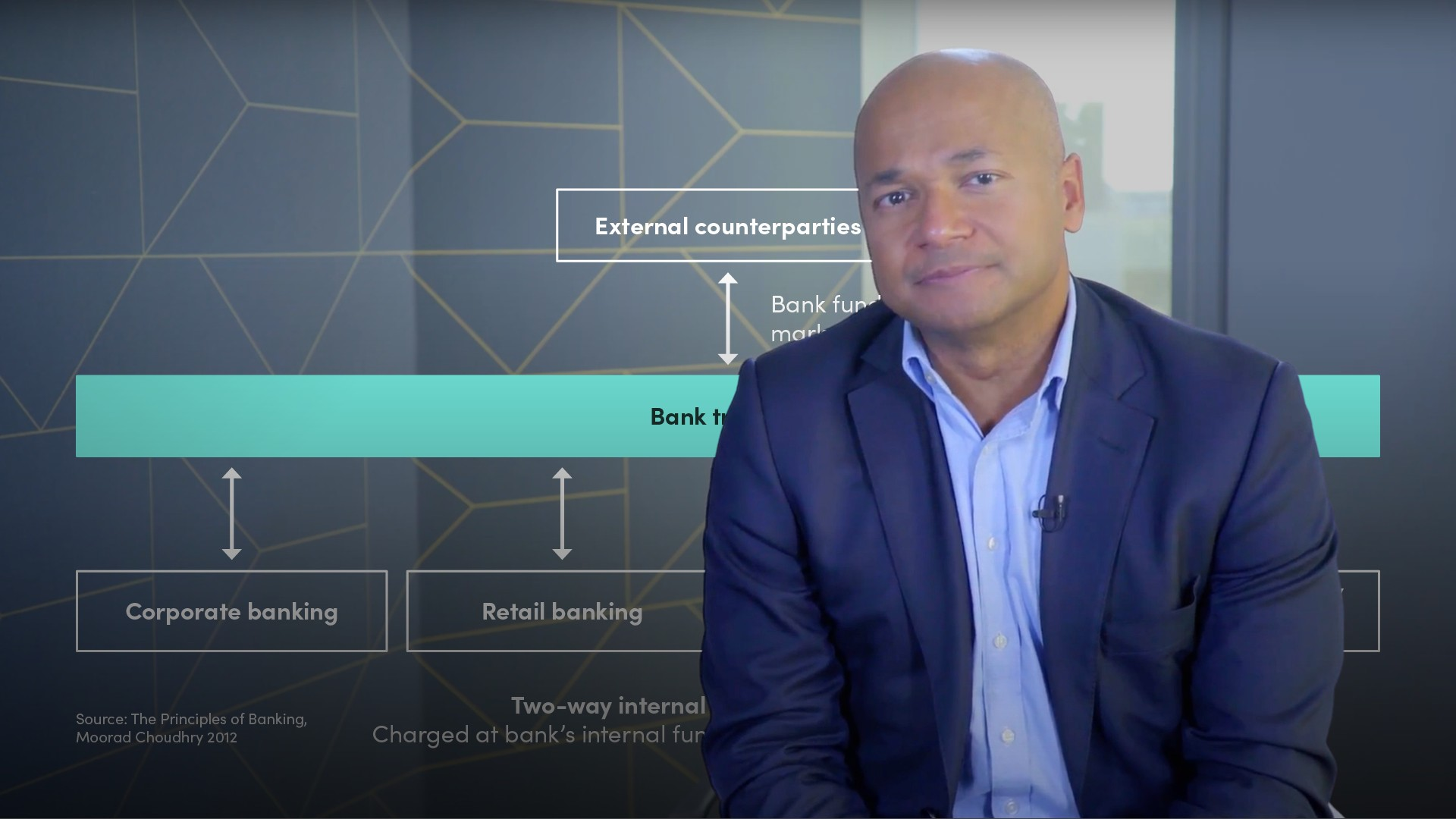16:5910:01

## Related videosRisk managementMarkets### Accredited by